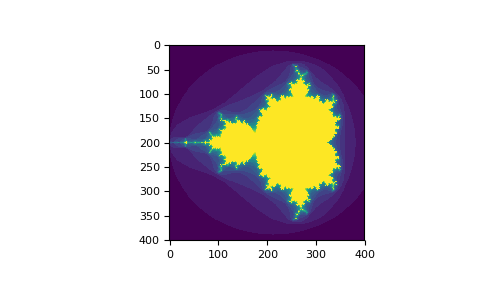# 布尔索引

Numpy给我们带来的最神奇的操作其实是布尔数组索引方法。

```>>> a = np.arange(12).reshape(3,4)
>>> b = a > 4
>>> b   # 通过比较运算，b变成了一个由布尔值组成的数组
array([[False, False, False, False],
[False,  True,  True,  True],
[ True,  True,  True,  True]])
>>> a[b]        # 生成一个由True值对应出来的一维数组
array([ 5,  6,  7,  8,  9, 10, 11])
```

```>>> a[b] = 0    #所有a中大于4的元素被重新赋值为0
>>> a
array([[0, 1, 2, 3],
[4, 0, 0, 0],
[0, 0, 0, 0]])
```

```>>> a[~b]
array([0, 1, 2, 3, 4])
>>> (a<4)|(a>7)
array([[ True,  True,  True,  True],
[False, False, False, False],
[ True,  True,  True,  True]])
>>> a[(a<4)|(a>7)]
array([ 0,  1,  2,  3,  8,  9, 10, 11])
>>> (a>3)&(a<8)
array([4, 5, 6, 7])
```

```>>> import numpy as np
>>> import matplotlib.pyplot as plt
>>> def mandelbrot( h,w, maxit=20 ):
...     """Returns an image of the Mandelbrot fractal of size (h,w)."""
...     y,x = np.ogrid[ -1.4:1.4:h*1j, -2:0.8:w*1j ]
...     c = x+y*1j
...     z = c
...     divtime = maxit + np.zeros(z.shape, dtype=int)
...
...     for i in range(maxit):
...         z = z**2 + c
...         diverge = z*np.conj(z) > 2**2     # 生成布尔数组
...         div_now = diverge & (divtime==maxit)  # 进一步处理
...         divtime[div_now] = i                  # 进行索引
...         z[diverge] = 2                        # 避免多次操作
...
...     return divtime
>>> plt.imshow(mandelbrot(400,400))
>>> plt.show()
``````>>> a = np.arange(12).reshape(3,4)
>>> b1 = np.array([False,True,True])
>>> b2 = np.array([True,False,True,False])
>>>
>>> a[b1,:]
array([[ 4,  5,  6,  7],
[ 8,  9, 10, 11]])
>>>
>>> a[b1]
array([[ 4,  5,  6,  7],
[ 8,  9, 10, 11]])
>>>
>>> a[:,b2]
array([[ 0,  2],
[ 4,  6],
[ 8, 10]])
>>>
>>> a[b1,b2]
array([ 4, 10])
```

```>>> a = np.arange(12).reshape(3,4)
>>> b1 = np.array([True,False,True])

>>> b1.nonzero()   # 调用nonzero方法，就相当于将值为True的下标索引拿出来，保存到一个新数组中
(array([0, 2], dtype=int64),)

>>> a[b1]
array([[ 0,  1,  2,  3],
[ 8,  9, 10, 11]])

>>> a[b1.nonzero()]             #可以看到，布尔数组索引等同于它的nonzero()数组索引的结果
array([[ 0,  1,  2,  3],
[ 8,  9, 10, 11]])

>>> b2 = np.array([True,False,True,False])

>>> b2.nonzero()
(array([0, 2], dtype=int64),)

>>> a[b2.nonzero()]
array([[ 0,  1,  2,  3],
[ 8,  9, 10, 11]])

>>> a[b1,b2]
array([ 0, 10])

>>> a[b1.nonzero(), b2.nonzero()]       # 同时使用两个布尔数组进行索引， 相当于用它们的nonzero()进行索引
array([[ 0, 10]])       # 也就是获取a,a。理解这点很重要！这时候从按行列索引，变成了按下标组合的索引方式。

# 要注意的是使用这种方法，b1和b2布尔数组的长度，必须相等或者可以广播。注意，这个广播机制很重要！
>>> b2 = np.array([True,False,True,True])

>>> b2.nonzero()
(array([0, 2, 3], dtype=int64),)

>>> a[b1, b2]
IndexError                                Traceback (most recent call last)
<ipython-input-26-c7d1e0872c96> in <module>
----> 1 a[b1, b2]

IndexError: shape mismatch: indexing arrays could not be broadcast together with shapes (2,) (3,)
#上面，长度2和长度3的两个索引数组无法广播，所以会出错。而下面长度1和2的两个数组，长度为1的可以广播，因此不会报错。

>>> b2 = np.array([False,False,True,False])

>>> b2.nonzero()
(array(, dtype=int64),)
>>> a[b1,b2]
array([ 2, 10])
```

#### 评论总数： 2>>> a[b1,b2] array([ 4, 10]) 这一步怎么来的？？ 求告知